Time: 3 Hours                                                                                                     Max. Marks: 100

NOTE: There are 9 Questions in all.

·      Question 1 is compulsory and carries 20 marks. Answer to Q. 1. must be written in the space provided for it in the answer book supplied and nowhere else.

·      Out of the remaining EIGHT Questions answer any FIVE Questions. Each question carries 16 marks.

·      Any required data not explicitly given, may be suitably assumed and stated.

Q.1       Choose the correct or best alternative in the following:                                         (2x10)

a.       The time taken by light emitted by phosphore to decay to its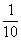th of its original intensity is called

(A)  persistence                                   (B)  resolution

(C)    Refresh rate                                 (D)  none of the above

b.      The electron beam is directed only to those parts of the screen where a picture is drawn, the display unit is operating as

(A)    Raster scan display                       (B)  random scan display

(C)  plasma display                              (D)  liquid crystal display

c.   An 8-bit plane frame buffer for a roaster scan screen with 1024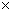1024 resolution requires ________memory bytes.

(A)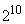(B)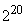(C)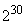(D)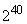d.   If the starting end point of a line is to the right of the terminating end point then (on x-y co-ordinate system)

(A)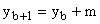(B)(C)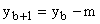(D)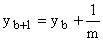e.   Transformation matrix for rotating an object about a point (10, 20) on the object through an angle of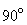in counter clockwise direction iswhere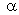is equals to

(A)     1                                                  (B)  -1

(C)  0                                                  (D)  10

f.    Bezier curve blending function can be defined as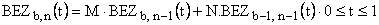The values of M and N are ________, ________ respectively.

(A)     t, (t-1)                                          (B)  b, (b-1)

(C)  (1-t), t                                          (D)  n, (n-1)

g.   The line with end points codes (1000) and (0001) in Cohen Sutherland algo is

(A)     visible                                          (B) invisible

(C)  partially visible                              (D) data incomplete.

h.   A fast and simple object space method for identifying the back faces of a polyhedron is based on

(A)    inside-outside test.                        (B) inside test.

(C) outside test.                                   (D) on the edge test.

i.    In midpoint subdivision algorithm the maximum number of subdivision is equal to

(A)   Precision of the number of bits used to represent the end points of the line

(B)   The no of edges of the polygon.

(C) system used to run the program.

(D) none of these.

j.    Oblique projection of an object with projection angle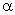such that tan=2 is called

(A)  cavalier projection                        (B)   cabinet projection

(C)  orthographic projection                 (D)   none of these

Answer any FIVE Questions out of EIGHT Questions.

Each question carries 16 marks.

Q.2     a.   How is computer graphics used in Computer aided design?                                 (4)

b.   What do you mean by aspect ratio? For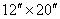VDU with 10241024 pixels, what is the aspect ratio?                                                                                                                         (3)

c.   Describe the technology of plasma panel display device.                                     (6)

d.   Differentiate between raster and random displays.                                               (3)

Q.3     a.   Describe the electromagnetic, electrical and acoustic implementation of digital tablets.                     (8)

b.   Given triangle with the vertices (1, 4), (5, 7) and (6,10). What would be the new co-ordinates of this triangle if it is rotated about the point (4, 7) through an angle of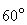.                                              (6)

c.   How can the zooming and panning be performed?                                              (2)

Q.4     a.   What are sound snags? Discuss briefly.                                                               (3)

b.   What do you understand by C1 and C2 continuity between two cubic Bezier segments? Derive the conditions for the same in terms of control point of the two cubic Bezier curve segments.     (8)

c.   Define B-spline. What do you mean by knot vector?                                           (5)

Q.5     a.   Write the steps of mid point subdivision algorithm.                                              (4)

b.   Briefly explain the two different representations of storing computer fonts.           (5)

c.   Fill the closed polygon with the vertices (5, 6), (5,12) (14,12), (14,6). Use scan line seed fill algorithm with (9,9) as seed. Fill only two scan lines.   (7)

Q.6     a.   What do you understand by light emitting and light-reflecting sources? Explain with the help of examples.                                                                (4)

b.   The operation tilting is defined as: Rotation about X-Axis followed by rotation about Y-axis. Give the transformation matrix for tilting in 3D.   (6)

c.   Describe briefly depth-buffer method for detecting visible surfaces.                     (6)

Q.7     a.   Write the perspective projection transformation matrix. What is the perspective projection of the point (1, 2, 3) on the plane z=6, if the projection reference point is (0, 0, 9)?                         (8)

b.   Explain Phong specular-reflection model.                                                            (6)

c.   Define the terms window and view port.                                                             (2)

Q.8     a.   Describe briefly the constructive solid-geometry method for generating solids using different techniques.                                                                 (8)

b.   Explain how the pixel positions are computed for a circle using Bresenhem’s algorithm.                   (8)

Q.9     a.   What do you mean by multimedia?  Explain how is it useful in our daily life.                        (5)

b.   How is sound stored in a computer?  Explain.                                                     (8)

c.   What is hypertext?  Where do we need it?                                                          (3)